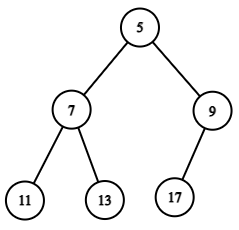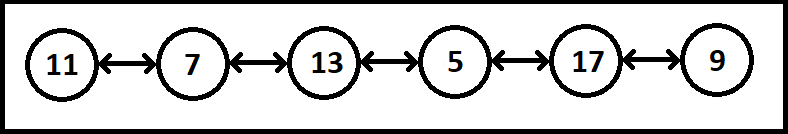# Binary Tree to Doubly Linked List

## Introduction

Binary Trees are a type of data structure where each data is stored as a node, and each node has two child nodes. The three primary traversal techniques are pre-order, in-order, and post-order.

Linked Lists are another type of data structure where data is stored in nodes, and each node is connected via links. A linked list can be singly linked or doubly-linked depending upon the number of links. The two pointers in a doubly-linked list are: next and previous.

## Problem Statement

Given a binary tree with nodes, convert it into a doubly-linked list.

Note: Store the inorder traversal of the given binary tree to the doubly linked list.

## Example

Sample InputExpected Output## Approach and Explanation

The implementation of the given problem is done in Java.

The step-by-step explanation is as follows -

• Binary Tree and Linked List are abstract data structures. This means that we need to create/define them so that we can use them.

• We create a constructor named TLL. It has three members- data(int), left(TLL), and right(TLL). The variable data contains the node’s value and left and right are the links that will connect the nodes.

• Once this is done, create a class that will do all our work and convert the binary tree into a linked list. Inside this class, have two global variables named root and head of the constructor type (TLL in our case) to perform the in-place conversion.

• Create a function that will recursively convert a Binary Tree node into a node of the Doubly Linked List. Name this function as createDLL().

• The variable root is for our binary tree, and the variable head is for our doubly linked list.

• The base case of the recursion is that if the root is null, we simply return and end the recursion. Else we recur for the right sub-tree first and set its value to the head of our linked list.

• We then check if the head is null or not. If it is not null, then the left side of the head contains the root. After assigning the value of root in the head, we traverse the left sub-tree.

• In the given implementation, there are two print functions. One of them is for the linked list, and the other is for the binary tree.

• In the function printLL(TLL head), we pass the head node of our linked list and print by traversing the whole list.

• In the function printBT(TLL root), we pass the root of our binary tree, perform level order traversal and print the tree level-wise.

• Inside our main() function, we create our binary tree object and pass some values for our nodes and then call the following three functions:
• printBT(): To print the binary tree given as the input.
• createDLL(): To create a doubly-linked list from the binary tree.
• printLL(): To print the doubly-linked list.

Recommended: Try to solve the problem first on our CodeStudio by clicking here Convert A Given Binary Tree To Doubly Linked List

## Java implementation

``````import java.util.*;

class TLL{
int data;
TLL left,right;

public TLL(int value){
data = value;
left = right = null;
}
}

public class BTreeDLL {
TLL root;

void createDLL(TLL root)
{
if (root == null)
return;
createDLL(root.right);

createDLL(root.left);
}

{
}
}

void printBT(TLL root){
if(root == null)
return;

while(true)
{
int nC = q.size();
if(nC == 0)
break;
while(nC > 0)
{
TLL node = q.peek();
System.out.print(node.data + " ");
q.remove();
if(node.left != null)
if(node.right != null)
nC--;
}
System.out.println();
}
}

public static void main(String[] args){

BTreeDLL tree = new BTreeDLL();
tree.root = new TLL(5);
tree.root.left = new TLL(7);
tree.root.right = new TLL(9);
tree.root.left.left = new TLL(11);
tree.root.left.right = new TLL(13);
tree.root.right.left = new TLL(17);

System.out.println("The Binary Tree is:");
tree.printBT(tree.root);

tree.createDLL(tree.root);

}
}``````

OUTPUT

``````The Binary Tree is:
5
7 9
11 13 17
The Linked List Formed: 11 7 13 5 17 9 ``````

## Complexities

### Time Complexity

We traverse our binary tree of n nodes once in the given implementation to create our doubly linked list. Thus time complexity is:

T(n) = O(n)

where n is the number of nodes.

### Space Complexity

In the given implementation, we use recursion to make our doubly linked list. Thus,

Space Complexity = O(h)

O(h) is the extra space for the recursion stack, where h is the height of the binary tree.

1. Are there other ways to convert a binary tree into a doubly-linked list?
Yes, there are a few more ways to convert a binary tree into a doubly-linked list. One of them is to perform in-order traversal, keep track of the visited nodes of our linked list and insert each visited node at the tail of our linked list.

2. Are these problems asked in coding interviews?

## Key Takeaways

In a nutshell, this article discussed the conversion of a binary tree to a doubly-linked list thoroughly. We saw the problem statement, an example, an approach to the problem, its Java implementation, and the time and space complexities. We concluded the article with a few FAQs.

Want to ace the coding rounds of big tech companies? Try our Attempt Unlimited Online Mock Test Series to start your preparation.
Learn various topics from Web Technologies, Programming Fundamentals, Data Structures, and Algorithms from our Library.

Happy Coding!